# Electronics and Communication Engineering - Exam Questions Papers

41.

Given L[f(t)] =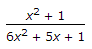and L[g(t)] =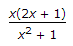also h(t) =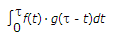, where, x =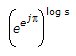then, L[h(t)] = __________ .

 A.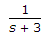B.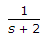C.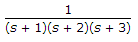D.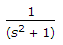Explanation:

Now, x =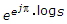log x = ejp x log S log x = y,

x = ey

log x = log Sejp

x = Sejp

x = S-1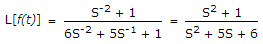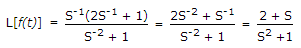L[f(t)] = L[f(t)] x L[g(t)].

42.

The voltage reflection coefficient on a 50 Ω line is 0.7∠30°.
The load impedance is

 A.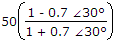B.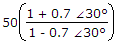C.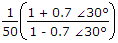D.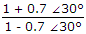Explanation: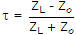.

43.

For finding the square root of N using Newton's iterative formula, the correct expression is:

 A.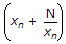B.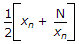C.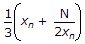D.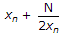Explanation: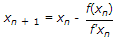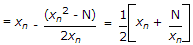f(xn) = (x2n - N).

44.

For a random variable x following the probability density function, p(x), shown in figure, the mean and the variance are respectively,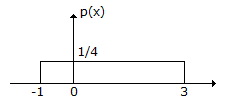A. 1/2 and 2/3 B. 1 and 4/3 C. 1 and 2/3 D. 2 and 4/3

Explanation:

The mean x is given by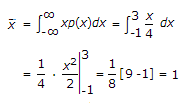and the variance is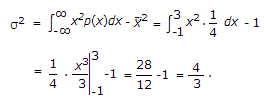45.

VR at t < T, t = ∞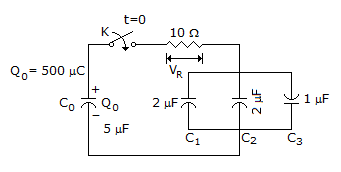A. 500e-40x104t, 0 B. 100e-40x103t, 0 C. 100e-40x106t, 0 D. 100e-40x104t, 0

Explanation:

At t = 0, C1, C2, C3 acts as short.

entire voltage will appear cross R.

At t increases the voltage across the resistance decrease exponentially.

T = RCnet = 10 x 2.5 x 10-6 = 25 μ sec

Initial voltage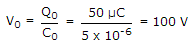VR = V0e-t/RC at t = 0 = 100

= 100 e-t/25x10-6 V.

VR = 100 e-40x104t V.

At t = ∞, VR = 0

The charge C0 will be distributed in C1, C2, C3 and no current will flow.

#### Current Affairs 2022

Interview Questions and Answers